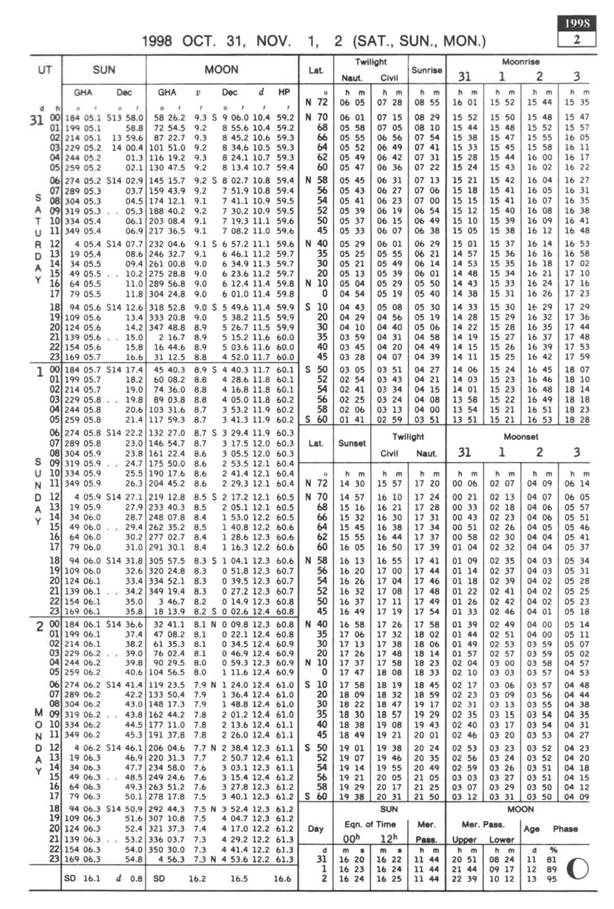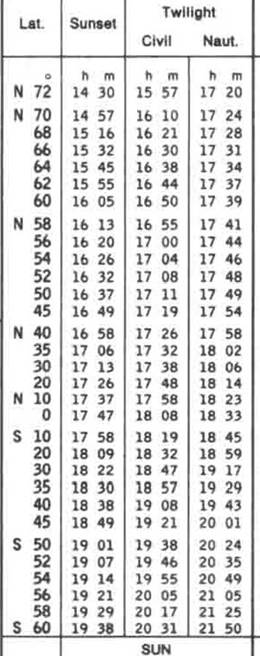Back to Nautical Solar Sys. Hour Angles PZX Triangles Dip Amplitude Day Ex Meridian Exercises Pole Star Naut. Almanac

Amplitude

Determination of the observed altitude of the sun when the true altitude is zero

The semi diameter is half the angular distance of a body as viewed from the Earth.

The altitude of the body’s centre above the rational horizon is the quantity required in nautical astronomy. Since we are unable to exactly see the centre of the Sun we take the altitude of the edge of the Sun nearest to the horizon and then the semi diameter as given in the Nautical Almanac is added to get the altitude of the centre of the Sun.

However since the Sun and all bodies are affected by the temperature and pressure when taking the refraction into account the Nautical Almanac has simplified the balance of the corrections into Total corrections. One page gives for 10˚-90˚ and the other page gives for 0˚-10˚.

Thus when we take an altitude as the sun is rising and the lower edge is just touching the horizon the actual body is well below the horizon, as shown below.

Date 31.10.1999

We assume the Height of Eye at 10 metres- Total Correction 0˚15’ – (-) 15.1’ (lower Limb – October to March)

 HOE 10m Sext. Alt. 20.7 IE 0 Obs. Alt. 20.7 Dip            (-) 5.6 App. Alt. 15.1 Total Corr.  (-) 15.1 True Alt. 0

We assume the Height of Eye at NIL- Total Correction 0˚15’ – (-) 15.1’ (lower Limb – October to March)

 HOE NIL Sext 15.1 IE 0 Obs. Alt. 15.1 Dip            (-) 0 App. Alt. 15.1 Total Corr.  (-) 15.1 True Alt. 0

From the above we see that to get the True Altitude as 0˚ we have to get the Observed Altitude as 15.1’ at sea level and in addition the height of eye would add some more.

The fact that it does not agree with the SD for that day is due to the refraction,

The Bearing Amplitude of a body is the arc of the horizon contained between the East point and the object when rising or between the West point and the object when setting.

It is also defined as the angle at the zenith between the prime vertical and the vertical circle of the body when on the horizon.

A body always rises and sets on a Northerly bearing when its declination is North and on a Southerly bearing when its declination is South,

However when the declination is 0˚ then the bearing is true East at rising and true West when setting. The amplitude therefore takes the name of the declination.

The azimuth at rising or setting is the complement of the amplitude, thus the Azimuth = (90˚ - amplitude).

The True amplitude of the sun (since the star is practically never visible while the exact moment of its rise) depends upon the solution of a right-angled spherical triangle.

Thus the formula:

Sine Amplitude = sine declination x secant Latitude.

The latitude is from the ships position, and the declination is got from the Nautical Almanac.

We can see therefore that an error in the Latitude would give a totally wrong amplitude and therefore a wrong compass error – since amplitude is an excellent means for obtaining the compass error.

Calculation of the Setting time of the Sun:

(same calculation also for the rising and the calculation of Civil Twilight)

Extracts from the Nautical Almanac

(see following sheet)Assume that a ship is in position:

Lat: 22˚00’N, Long: 82˚00’E

Required to find the Sunset on the 31st Oct 1999.

First open the page in the NA to the date and then look up the Sunset column for the Sun. The column is as shown.

Since we are in the NH look at N20 and also at N30 interpolate to get the h and m for Lat 22˚ it would be:17 23.4 or roughly 17h 23m. Note that the time is reducing from 20˚ to 30˚. Now convert the Longitude into time and subtract from the same to get the GMT of the Sunrise.

After doing that add the time zone kept by the ship and get the LMT of the Sunset.